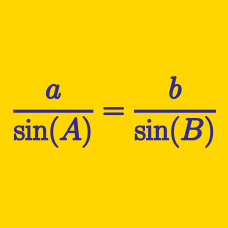Geometry

# Triangle Solving Warmup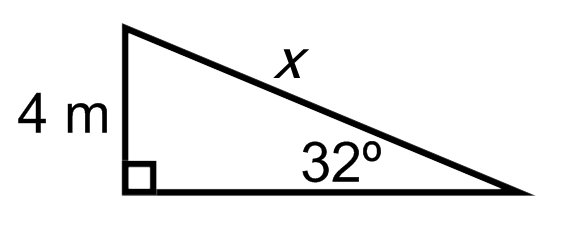What is $x?$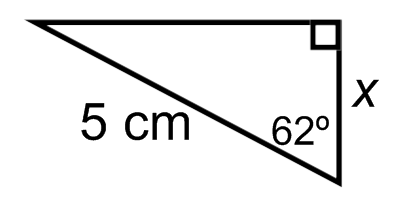What is $x$?Which of these is equivalent to $\cos(a)$?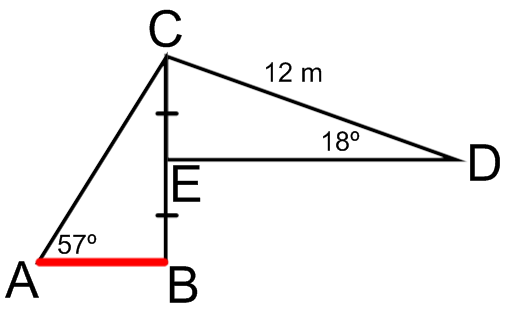What is the length of $\overline{AB}?$ (marked in red)

Earlier, we used angles to help solve for side lengths of right triangles. Let's see if we can reason with side lengths to solve for angles.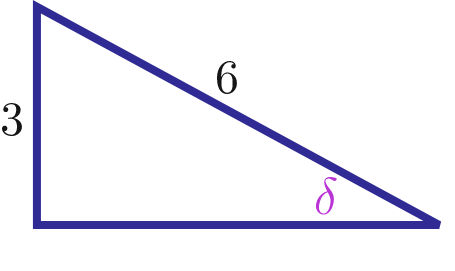What is $\delta$ in radians?

×